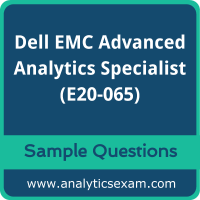# Dell EMC Advanced Analytics Specialist (E20-065) Certification Exam Sample QuestionsHere are the sample questions which will help you be familiar with Dell EMC Advanced Analytics Specialist for Data Scientists (E20-065) exam style and structure. We encourage you to try our Demo Dell EMC Advanced Analytics Specialist Certification Practice Exam to measure your understanding of exam structure in an environment which simulates the Dell EMC Certified Specialist - Data Scientist - Advanced Analytics (DCS-DS) Certification test environment.

To make your preparation more easy for Dell EMC Certified Specialist - Data Scientist - Advanced Analytics (DCS-DS) (E20-065) exam, we strongly recommend you to use our Premium Dell EMC Advanced Analytics Specialist Certification Practice Exam. According to our survey with certified candidates, you can easily score 85% in your actual Dell EMC Certification exam if you can score 100% in our premium Dell EMC Advanced Analytics Specialist Certification practice exams.

## Dell EMC E20-065 Sample Questions:

01. You conduct a TFIDF analysis on 3 documents containing raw text and derive TFIDF ("data", document y) = 1.908. You know that the term "data” only appears in document 2.
What is the TF of “data" in document 2?
a) 2 based on the following reasoning: TFIDF = TF1DF = 1 908 You then know that IDF will equal LOG (32)=0.954 Therefore, TFIDF=TF*0.954 = 1.908 TF will then round to 2
b) 4 based on the following reasoning: TFIDF = TF1DF = 1.908 You then know that IDF will equal LOG (3/1 )=0.477 Therefore, TFIDF=TF'0 477 = 1.908 TF will then round to 4
c) 6 based on the following reasoning: TFIDF = TF1DF = 1.908 You then know that IDF will equal 3/1=3 Therefore, TFIDF=TF/3 = 1.908 TF will then round to 6
d) 11 based on the following reasoning: TFIDF = TF1DF = 1908 You then know that IDF will equal LOG(3/2)=0.176 Therefore, TFIDF=TF"0.176 = 1.908 TF will then round to 11

02. Which scenario is a proper use case for multinomial logistic regression?
a) A marketing firm wants to estimate the personal income of a group of potential customers. Using inputs such as age, education, marital status, and credit card expenditures, a data scientist is building a model that will estimate a person's income
b) A logistic distribution company wants to minimize the distance traveled by its delivery trucks. A data scientist is building a model to determine the optimal route for each of tis trucks
c) To improve the initial routing of a loan application, a financial institution plans to classify a loan application as Approve, Reject, or Possibly_Approve. Based on the company's historical loan application data, a data scientist is building a model to assign one of these three outcomes to each submitted application.
d) A manufacturer plans to determine the optimal number of workers to employ in an assembly line process. Utilizing the observed distributions of the task durations of each process step, a data scientist is building a model to mimic the interactions and dependencies between each stage in the manufacturing process.

03. You are analyzing written transcripts of focus groups conducted on product X. You approach is to use TF-IDF for your analysis.
What combination of TF-IDF scores should you examine to ensure you only report on the most important terms?
a) High TF score and high DF score
b) High TF score and high IDF score
c) High TF score and low IDF score
d) Low TF score and low DF score

04. Why would a company decide to use HBase to replace an existing relational database?
a) It is required for performing ad-hoc queries.
b) Varying formats of input data requires columns to be added in real time.
c) The company's employees are already fluent in SQL.
d) Existing SQL code will run unchanged on HBase.

05. What is a characteristic of stop words?
a) Meaningful words requiring a parser to stop and examine them
b) Don't occur often in text
c) Used in term frequency analysis
d) Include words such as "a", "an", and "the"

06. Which problem type is best suited for simulation?
a) One with a few. non-random input variables
b) One that has a closed-form solution
c) One with numerous, non-random Input-variables
d) One that compares "what-if scenarios

07. According to Metcalfe's law, what is true about the value of a network?
a) Proportional to the number of edges
b) Proportional to the logarithm of the number of edges
c) Unrelated to the number of edges
d) Proportional to the square of the number of edges

08. You develop a Python script "logisticpy" to evaluate the logistic function denoted as f(y) for a given value y that includes the following Pig code:
Register 'logistic.py' using jython as udf; z = FOREACH y GENERATE \$0, udf.logistic (\$0); DUMP z;
What is the expected output when the Pig code is executed?
a) 0
b) Jython is not a supported language
c) Value of f(y) for ally
d) Tuples (y, f(y))

09. Which scenario would be ideal for processing Hadoop data with Hive?
a) Unstructured data; batch processing
b) Structured data, real-time processing
c) Structured data; batch processing
d) Unstructured data; real-time processing

10. What are key characteristics of Random Graphs?
a) Low clustering coefficients; high network diameters
b) Low clustering coefficients; small network diameters
c) High clustering coefficients; high network diameters
d) High clustering coefficients; small network diameters